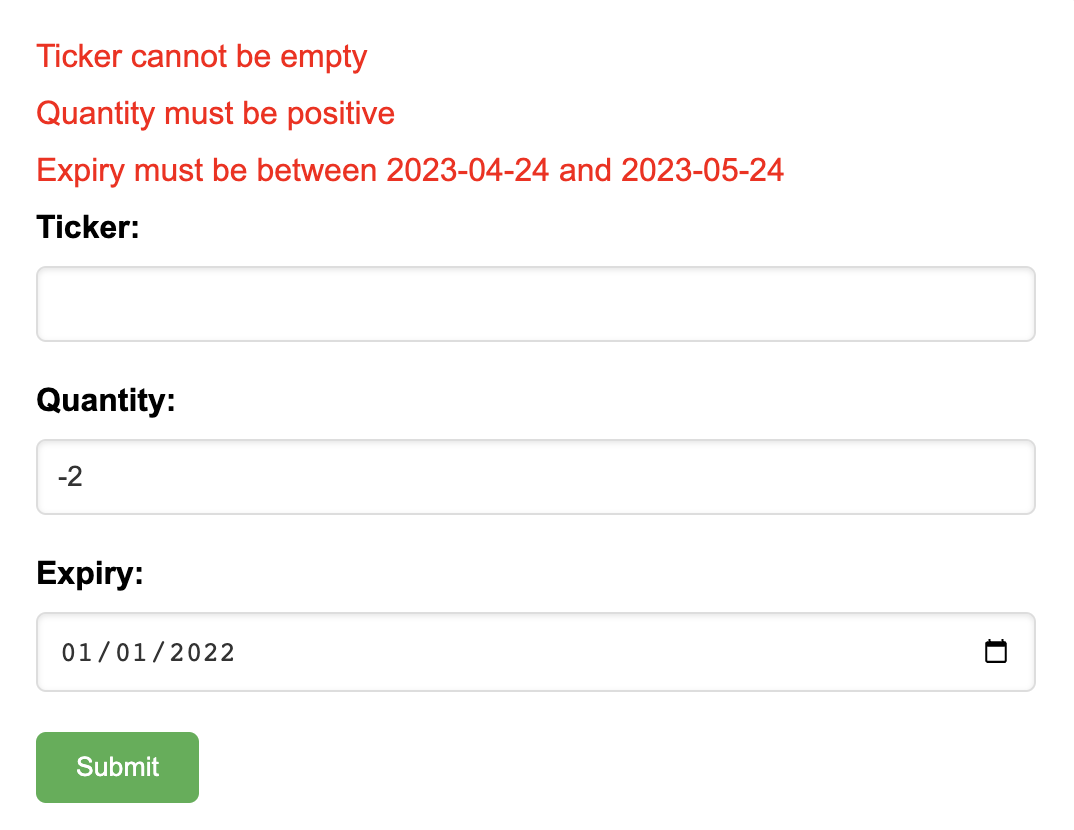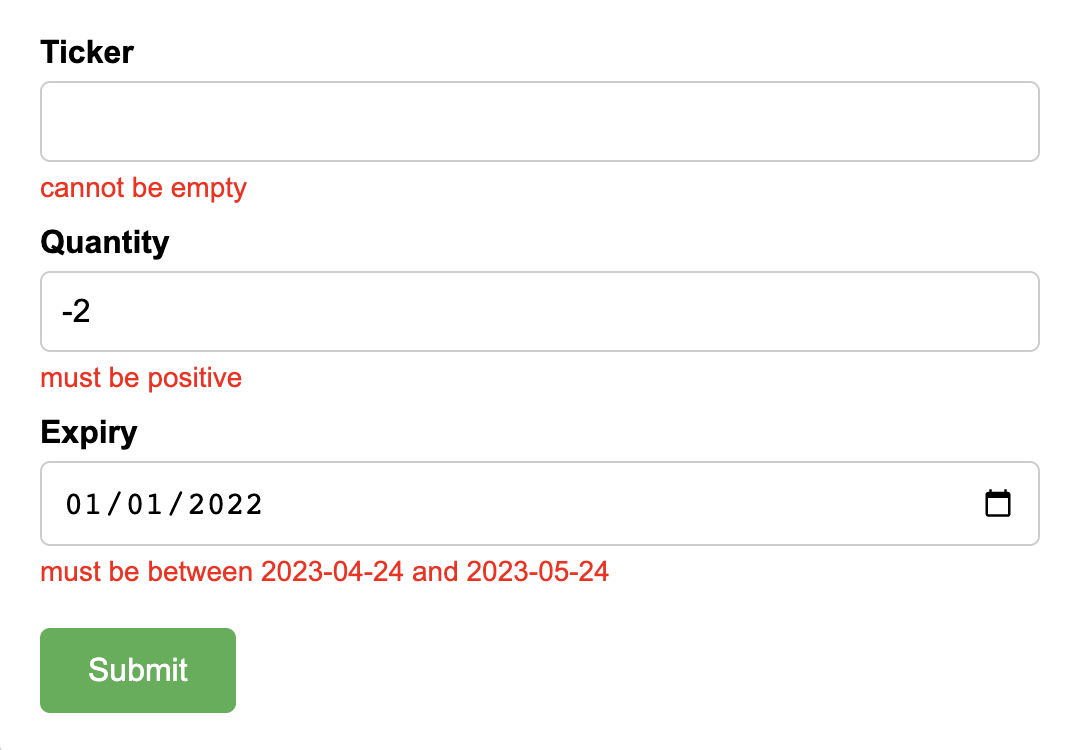# Accumulate errors in Scala with typelevel cats

Julien Truffaut

2nd May 2023

When it comes to handling errors, the go-to strategy is to stop all computations after encountering the first error. This is typically achieved through the use of exceptions. While this approach works in most cases, there are times when it isn't ideal. For instance, when receiving a user request, it is preferable to return all the errors at once and allow the users to fix them in one go. In this blog post, I'll delve into such a scenario and explore a concrete example using Scala 3 and the Cats library.

You can find all the code samples in the following github repository.

## The problem

Let’s say that we work for a financial institution which receives orders to buy or sell financial products. Here is a simple request:

```case class CreateOrderRequest( ticker : String, quantity: Long, expiry : Option[LocalDate], )```

The ticker is an identifier for the financial instrument. The quantity represents the number of instruments desired. Finally, the expiry is an optional field which tells us when the request is valid.

We want to verify the following three constraints:

1. The ticker must not be empty
2. The quantity must be positive
3. The expiry must either be empty or no later than a month in the future.

Let’s implement those rules:

```def validateTicker(ticker: String): Either[String, String] = if(ticker.isEmpty) Left("Ticker cannot be empty") else Right(ticker) def validateQuantity(quantity: Long): Either[String, Long] = if(quantity <= 0) Left("Quantity must be positive") else Right(quantity)```

For the expiry, we may want to introduce a custom enumeration to make it explicit that `None` means that the request doesn't expire.

```enum Expiry { case Never case ValidUntil(date: LocalDate) } def validateExpiry(optExpiry: Option[LocalDate], today: LocalDate): Either[String, Expiry] = optExpiry match { case None => Right(Expiry.Never) case Some(expiry) => val min = today val max = today.plusMonths(1) if (expiry.isBefore(min) || expiry.isAfter(max)) Left(s"Expiry must be between \$min and \$max") else Right(Expiry.ValidUntil(expiry)) }```

Once we have implemented these validation rules, we can combine them using a for-comprehension:

```def validateOrder(request: CreateOrderRequest, today: LocalDate): Either[String, Order] = for { ticker <- validateTicker(request.ticker) quantity <- validateQuantity(request.quantity) expiry <- validateExpiry(request.expiry, today) } yield Order(ticker, quantity, expiry) ```

Now, let’s give it a try:

```validateOrder( CreateOrderRequest( ticker = "AAPL", quantity = 10, expiry = None, ), LocalDate.of(2023,4,24), ) // res = Right(Order("AAPL", 10, Expiry.Never))) validateOrder( CreateOrderRequest( ticker = "AAPL", quantity = -2, expiry = None, ), LocalDate.of(2023,4,24), ) // res = Left("Quantity must be positive"))```

So far so good. However, if the request contains multiple errors, we only see the first error concerning the invalid ticker and we don't realise that the quantity and the expiry fields are also invalid.

```validateOrder( CreateOrderRequest( ticker = "", quantity = -2, expiry = Some(LocalDate.of(2022, 1, 1)), ), LocalDate.of(2023,4,24), ) // res = Left("Ticker cannot be empty"))```

We only see the first error because a for-comprehension is by nature sequential, meaning that it only executes the next line if the previous line has been completed with some data. Therefore, if we want to accumulate errors, we can’t use a for-comprehension or `flatMap`,we need to use another method. But which one?

## Solution 1: parMapN

Typelevel Cats comes to the rescue! Cats is a functional library with lots of useful data types and functions which complement the Scala standard library very well.

All the code for this section can be found here

We are going to replace the for-comprehension with `parMapN`, which is going to merge all the errors together if there are any.

```import cats.implicits.* def validateOrder(request: CreateOrderRequest, today: LocalDate): Either[String, Order] = ( validateTicker(request.ticker), validateQuantity(request.quantity), validateExpiry(request.expiry, today), ).parMapN( (ticker, quantity, expiry) => Order(request.id, ticker, quantity, expiry) )```

`validateOrder` produces the same results when the request is valid or if it contains just one error. The only difference is when the request contains multiple errors:

```validateOrder( CreateOrderRequest( ticker = "", quantity = -2, expiry = Some(LocalDate.of(2022, 1, 1)), ), LocalDate.of(2023,4,24), ) // res = Left("Ticker cannot be emptyQuantity must be positiveExpiry must be between 2023-04-24 and 2023-05-24")```

Now we see all the error messages. Unfortunately, they are all squashed together into a single String, without punctuation or even spaces between them. A better approach would be to put the error messages into a data structure such as a `List`. This will allow us to choose how to display those errors later on.

## Solution 2: parMapN with List

Let’s update our three validation functions so that they return a `List` of errors.

```def validateTicker(ticker: String): Either[List[String], String] = if(ticker.isEmpty) Left(List("Ticker cannot be empty")) else Right(ticker)```

We also do a similar update for `validateQuantity` and `validateExpiry`. You can find all the code for this section here.

We also need to change the error type of `validateOrder` to `List` but otherwise the body of the function stays the same:

```def validateOrder(request: CreateOrderRequest, today: LocalDate): Either[List[String], Order] = ( validateTicker(request.ticker), validateQuantity(request.quantity), validateExpiry(request.expiry, today), ).parMapN( (ticker, quantity, expiry) => Order(request.id, ticker, quantity, expiry) )```

And now it works as expected, as you can see below:

```validateOrder( CreateOrderRequest( ticker = "", quantity = -2, expiry = Some(LocalDate.of(2022, 1, 1)), ), LocalDate.of(2023,4,24), ) // res = Left(List( // "Ticker cannot be empty”, // “Quantity must be positive”, // “Expiry must be between 2023-04-24 and 2023-05-24" //))```

This will allow us to show all the error messages to our user:This is good but it would be even better if we could display the error messages next to the field causing the error! Something like the image below.In order to do this, we would need to associate each error message with the ID of a field. Let’s give it a try.

## Solution 3: parMapN with a Map

Once again, let’s update our validation functions so that they package the errors into a `Map`.

```type FieldId = String type OrderErrors = Map[FieldId, List[String]] def validateTicker(ticker: String): Either[OrderErrors, String] = if(ticker.isEmpty) Left(Map(“ticker” -> "cannot be empty")) else Right(ticker)```

Similarly for `validateQuantity`,`validateExpiry` and `validateOrder`. You can find all the code for this section here.

Now, let’s run the code with a request containing multiple errors:

```validateOrder( CreateOrderRequest( ticker = "", quantity = -2, expiry = Some(LocalDate.of(2022, 1, 1)), ), LocalDate.of(2023,4,24), ) // res = Left(Map( // “ticker” -> List("cannot be empty”), // “quantity” -> List(“must be positive”), // “expiry” -> List(“must be between 2023-04-24 and 2023-05-24") //))```

I find it quite impressive that we only needed to change the error type and then `parMapN` automatically combined the errors together! How did it work? Is `parMapN` overloaded to support common error types such as `String`, `List` and `Map`, or is it more generic?

## A peek behind the scenes

`parMapN` is a generic method which works on all error types as long as we can “squash” its values together. In practice, this means that the error type needs to have an implicit instance of the class `Semigroup`. It may sound complicated, but it is actually very simple to define. Here is an example for `String`:

```import cats.Semigroup given Semigroup[String] = new Semigroup[String] { def combine(x: String, y: String): String = x + y }```

We didn’t need to implement it for `String`, `List` or `Map` because the Cats library already did it for the most common types of the standard library. A simple way to test if an instance exists is to use the method `sumon`.

```summon[Semigroup[String]].combine("Hello", "World") // res = “HelloWorld” summon[Semigroup[Map[String, List[String]]]].combine( Map("id1" -> List("aaa", "bbb"), "id2" -> List("ccc")), Map("id2" -> List("ddd") , "id3" -> List("eee")), ) // res = Map( // "id1" -> List("aaa", "bbb"), // "id2" -> List("ccc", "ddd"), // "id3" -> List("eee"), // )) summon[Semigroup[UUID]].combine(UUID.randomUUID(), UUID.randomUUID()) // error: No given instance of type cats.kernel.Semigroup[java.util.UUID] was found for parameter x of method summon in object Predef```

As you can see, Cats has implemented a `Semigroup` instance for `String` and `Map` but not for `UUID` as there are no meaningful ways to combine `UUID`s together.

So if you want to accumulate errors in a custom type, you will need to define its own instance of `Semigroup`. You can find an example here.

## Bonus: validate a collection

We saw that `parMapN` works great to combine a few errors together, but what if the number of validations is unknown at compile time? For example, let’s say that we receive a batch of `CreateOrderRequest` to process, how can we validate all the requests and return all the errors for each request to the end user?

`def validateOrders(requests: List[CreateOrderRequest], today: LocalDate)`

We can’t use `parMapN` here because we don't know how many elements are in the `List`. In this case, we need to use another method from Cats: `parTraverse`.

```import cats.implicits.* def validateOrders(requests: List[CreateOrderRequest], today: LocalDate): Either[OrderErrors, List[Order]] = requests .parTraverse(request => validateOrder(request, today))```

Let’s give it a try with two invalid requests:

```validateOrder( CreateOrderRequest( ticker = "AAPL", quantity = -2, expiry = None, ), CreateOrderRequest( ticker = "", quantity = -2, expiry = Some(LocalDate.of(2022, 1, 1)), ), LocalDate.of(2023,4,24), ) // res = Left(Map( // “ticker” -> List("cannot be empty”), // “quantity” -> List(“must be positive”, “must be positive”), // “expiry” -> List(“must be between 2023-04-24 and 2023-05-24") //))```

It does work, but we don’t know which error corresponds to which order. For example, we see that the ticker shouldn’t be empty but we don’t know if this refers to the first or second request. There are a few ways to solve this problem.Here, I suggest to introduce a unique identifier for orders and assign an `OrderId` to each `OrderErrors`:

```import cats.implicits.* case class OrderId(value: String) // or UUID type MultipleOrderErrors = Map[OrderId, OrderErrors] def validateOrders( requests: List[CreateOrderRequest], today : LocalDate, ): Either[MultipleOrderErrors, List[Order]] = requests .parTraverse(request => validateOrder(request, today) .leftMap(orderError => Map(request.id -> orderError)) )```

Let’s re-run the same example with two invalid requests:

```validateOrder( CreateOrderRequest( id = OrderId("1111"), ticker = "AAPL", quantity = -2, expiry = None, ), CreateOrderRequest( id = OrderId("2222"), ticker = "", quantity = -2, expiry = Some(LocalDate.of(2022, 1, 1)), ), LocalDate.of(2023,4,24), ) // res = Left(Map( // OrderId("1111") -> Map( // FieldId.quantity -> List("must be positive"), // ), // OrderId("2222") -> Map( // FieldId.quantity -> List("must be positive"), // FieldId.expiry -> List("must be between 2023-04-24 and 2023-05-24"), // FieldId.ticker -> List("cannot be empty"), // ), // ))```

Perfect, this time we have all the necessary information to display the errors to our user!

To summarize, we've explored the limitations of for-comprehension when it comes to accumulating errors and learned about two powerful methods from the Cats library: `parMapN` and `parTraverse`. These methods offer a generic solution that works with any error type equipped with a `Semigroup` instance. We've also seen that Cats defines those instances for common types such as `String`, `List`, and `Map` and it is very easy to create our own instance when using a custom error type.

I hope this post has been informative and useful. Please feel free to share your thoughts on the reddit or suggest topics you'd like me to cover in future posts. Thank you for reading!